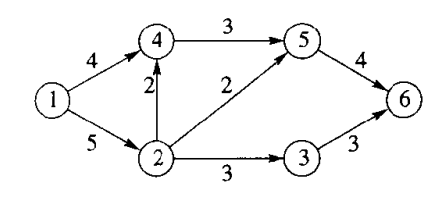# 最大流算法

## 最大流### 打标签算法(LABELING ALGORITHM)

#### 具体步骤

1. 定义一个集合 $$N$$ ，其中用于记录当前已经加入的点的集合，最开始将源点 1 加入 $$N$$
2. 将最新加入的元素进行延拓至所有可抵达的，且不属于$$N$$中的元素 (先后顺序参照序号) ，对于有向边$$e_{ij}$$，如果$$e_{ij}\gt0$$，则路径可通 (如果方向和图中路径相反，则考虑虚边是否大于零)，第一次则将 1 延拓至 2 4，并且将其分别标记上$$[5,1] [4,1]$$ (格式为[可以进入的最大流量, 从哪一个点延拓至该点])
3. 将新加入的点加入到 $$N$$
4. 回到步骤 2，直到 (假设汇点序号为 6) $$6\in N$$，记录此时最大的流量 $$f$$
5. 回溯延拓至汇点的路径，将其对应的实边 $$e_{ij}=e_{ij}-f$$，虚边$$e_{ji}=e_{ij}+f$$
6. 回到步骤 1 ，直到无法有路径延拓至汇点

Hyiker Hu

2020-12-16

2021-09-26